See You At ITEXPO & The #TechSuperShow | February 11-14, 2020 | Fort Lauderdale, Florida REGISTER TODAY.
SUBSCRIBE TO TMCnet

## TMC NEWS

Region Stability for a Class of Networked Control Systems [Sensors & Transducers (Canada)]
 [March 12, 2014]

# Region Stability for a Class of Networked Control Systems [Sensors & Transducers (Canada)]

(Sensors & Transducers (Canada) Via Acquire Media NewsEdge) Abstract: The stability analysis for Networked Control Systems (NCSs) has been one of the main research focuses in academia for many years. Some preliminary results have been derived in the existing literature. In this paper, the region stabilization is researched for a class of NCSs with uncertainties and random time-delays. The sufficient condition for feasibility is provided in the presence of communication constraints. And the maximum allowable delay is presented in term of linear matrix inequalities (LMIs) approach, which can be the optimization problem of computation the time-delay lower bound. The efficacy and feasibility of the proposed methodology is shown by presenting simulation results. Copyright © 2014IFSA Publishing, S. L.

Keywords: Networked control systems (NCSs), Region stability, Delay, Free-weighting matrix, LMI.

(ProQuest: ... denotes formulae omitted.) 1. Introduction Networked control systems (NCSs) have been one of the main research focuses nowadays, where the control loop is closed through a shared band limited network . NCSs can bring many new advantages, such as low cost, high reliability, ease installation and maintenance etc. Therefore, it has been widely applied to many industrial control systems, such as, manufacturing plants, vehicles, aircraft, and spacecraft [1-3]. But the insertion of the digital communication network can lead to timedelay, which can degrade a system's performance and even cause system instability . How to make the system stability is the main important problem for all researchers [4-17, 20, 21].

Hu et al.  researched the robust stabilization problem for a class of nonlinear discrete-time NCSs based on Takagi-Sugeno (T-S) fuzzy models. In , an event-triggering scheme for discrete-time NCSs was proposed. And by using a delay system approach, the sufficient conditions for the existence of an admissible guaranteed cost controller were studied. The performance analysis for a class of SISO networked control system with signal-to-noise ratio constraint is presented in reference  by using stabilizing compensators. Luan, Li, Wang, Sun and Huang et al. [7, 8, 11, 12, 15] considered the Hco controller design for NCSs with stochastic time-delay. By using new Linear Matrix Inequality (LMI) method, they obtained the sufficient condition for the asymptotical stability. Bauer et al.  provided mathematical models transformed NCSs into suitable hybrid systems formulations, and constructed Lyapunov functions using SOS techniques to analyze the stability of NCSs.

Among these reported results, time delay is the most important challenging problems. In this paper, our interest is also considering the region stabilization for uncertain NCSs with random timedelay. In order to reduce the conservatism, Lyapunov stability theory and LMI methodology are used to derive the sufficient condition. Notice that the result proposed can be easily extend to other multiple timedelays case.

The remainder of this paper is organized as follows. In Section 2, the structure and the mathematical model of uncertainty NCSs with timedelays is introduced. In Section 3, the imposed stabilization criterion is analyzed. And the sufficient condition with the maximum allowable transfer interval for convergence of the proposed method is derived. The efficacy and feasibility of the proposed methods is shown by presenting simulation results in Section 4. Finally conclusion remarks are presented in Section 5.

2. Problem Formulations Consider the plant with disturbance given by the following Equations.

... (1) where u(t)eRm , x(t)eR" , y(t) e Rr represent input vector, state vector and output vector respectively. The parameters A , B , C are known constant matrices with proper dimensions. AA, AB are assumed to be norm bounded and satisfy the following condition: ... (2) ... (3) where D , Ea , Eb denote the structure of uncertainty, F(t) is an unknown time-varying matrix with Lebesgue measurable elements. I represents the identity matrix with appropriate dimension.

Without loss of generality, we make the following assumptions [13, 16, 17]: 1) All the sensors are clock-driven, T is the sampling period, the controllers and the actuators are event-driven. u(t) = 0 before the first control signal reaches the plant.

2) The data is transmitted with one data packet through the communication network. That is, the data is transmitted with one data packet through communication network at every sampling period.

3) Time-delay is the only influence factor. And there are no loss data in the system.

Then by using the real memory-less state feedback control methodology u(t) and zeroth-order hold in Equation (1) with the following equation, such as: ... (4) If we only consider the effect of time-delay, then system (1) with Equation (4) can be rewritten as [14, 15].

... (5) where Tx is time-delay, which denotes the time from the instant when the sensors sample data to the instant when actuators transfer data to the plant.

According to Equation (2), system (5) can be rewritten as follows ... (6) In order to get the region stabilization criterion, Taking the state transformation x(í) = e~alTj(t), t> 0, then the functional differential Equation (7) can be given.

... (7) It is easy to know that the uniform asymptotic stability for system (7) can guarantee the a-uniformly asymptotical stable of system (6).

3. Main Results Before the development of the main result, the following lemmas could be used.

Lemma 1  (Schur complement theorem): Given the symmetric matrix S = ST.

... (8) where Sn <=Rrxr , SX2, S2X, S22 are real matrices with proper dimensions. Then the following three conditions are equivalent.

1) 51 <0 2) SnSl2S22SX2 < 0 and S22 < 0 3) S22-Sf2S;l'Sl2<0 and Sxx < 0 Lemma 2 : Given the symmetric matrix Q = QT , and the constant matrix H , E with appropriate dimension, if F(t) is an uncertain matrix function with Lebesgue measurable elements and satisfies FT (t)F(t) < /, in which / denotes the identity matrix of appropriate dimension. Then ... (9) If and only if there exists positive constant S > 0 such that ... (10) In the following, the sufficient a-uniformly asymptotical condition for convergence is given of system (6).

Theorem 1: Considering system (6), given positive constant r(0<r,<r) and positive constan £ . Then system (6) is CC -uniformly asymptotical stable if there exist symmetric constant matrix P> 0 , Q> 0, Z > 0 , matrix X , Nx, N2 with proper dimensions such that ... (12) where * denotes the symmetric terms in symmetric matrix and ...

Proof: Construct the Lyapunov-Krasovskii functional candidate is presented as follows : ... (13) where P and Q are the symmetric positive matrices.

1) From the Leibniz-Newton formula, Equation (14) is given.

... (14) Then the following equation (15) is true for any Free-Weighting Matrices Nx and N2 with appropriate dimensions.

... (15) 2) Give the symmetric positive matrices X as follows: ... (16) Then the equation (17) can be derived.

... (17) where ...

3) Calculating the derivative of V(t) in Equation (13) along the solutions of Equation (7), we have ... (18) Adding the left side of Equation (15) and Inequality (17) into it, Equation (19) can be given.

... (19) where ...

It can be proved that if the following matrix Inequality (20) holds, then system (6) is proofed a - uniformly asymptotical stable by the LyapunovKrasovskii functional theorem.

... (20) By the Schur complement theorem, and according to Inequality (20), Equation (21) is given.

... (21) Then rearranging inequality (20) by using Equation (21), Equation (22-23) can be derived.

... (22) -rZ ... (23) where ...

Using Lemma 2, the following Inequality (24-25) can be presented from Inequality (23).

... (24) ... (25) Now, using Lemma 1 (Schur complement theorem) to simplify and rearrange (25), Inequality (26) can be derived.

... (26) So we can see inequality (26) is the same as inequality (11) in Theorem 1.

The proof of Theorem 1 is completed.

4. Numerical Example In this section, the effectiveness of the proposed a -uniformly asymptotical stability with delaydependent of NCSs is demonstrated by numerical simulations.

Consider the plant with uncertainty as follows ...

where ...

For the convenience of investigation, the single packet transmission method is used facilitate the design and reliability. By using the real memory-less state feedback controller is described as follows: ...

When a = 0.2, by Theorem 1 in the paper, the Maximum Allowable Transfer Interval (MATI) which guarantees the stability of NCSs with uncertainty is rmax = 380 ms . However, using the Theorem 1 in reference , the maximum value of time-delay is rmax = 257 ms < 380 ms . Therefore, Theorem 1 can improve the result of reference  and has less conservation. The simulation results are shown in the flowing figure (*o = [o l -0.5 0.5]) * As can be seen from the simulation results (Figs. 1 and 2), two experiments are contributed to test the CC -uniformly asymptotical stability for a class of NCSs when A4*0 , Aß*0 and A4=0 , AB = 0 respectively. The dynamical behavior of the NCSs is converging to zero in less time. By simulating the plant, we successfully improve the system control performance and reduce the conservation.

6. Conclusions In this paper, the method of analysis the region stability condition for NCSs with parameter uncertainties and random delays has been proposed. Based on Lyapunov stability theory, the imposed a - stability condition and the MATI condition for systems is presented by introducing some free-weighting matrices and linear matrix inequalities (LMIs) which can be selected properly to lead to much less conservative results. But how to make the results extended to satisfy other NCSs with data packets lost is one of the most important issues to be investigated in the future [20-21].

Acknowledgements This work was supported by National Natural Science Foundation of China under grants 61203033, 61271377 and 61172131, 2013 National Student Innovation, Entrepreneurship, and Training Plan Foundation of China under grant 201310363054, and Anhui Provincial Natural Science Foundation under grants 1208085QF124 and 1208085MF115.

References  . R. A. Gupta, Mo-Yuen Chow, Networked control system: overview and research trends, IEEE Transactions on Industrial Electronics, Vol. 57, Issue 7,2010, pp. 2527-2535.

 . Wang Feiyue, Liu Derong, Networked control system theory and applications, Springer-Verlag London Limited, 2008.

 . T. C. Yang, C. Peng, D. Yue, M. R. Fei, New study of controller design for networked control systems, IET Control Theory & Applications, Vol. 4, Issue 7, 2010, pp. 1109-1121.

 . Hu Songlin, Zhang Yunning, Yin Xiuxia, D. Zhaoping, T-S fuzzy-model-based robust stabilization for a class of nonlinear discrete-time networked control systems, Nonlinear AnalysisHybrid Systems, Vol. 8,2013, pp. 69-82.

 . S. Hu, X. Yin, Y. Zhang, E. G. Tian, Event-triggered guaranteed cost control for uncertain discrete-time networked control systems with time-varying transmission delays, IET Control Theory and Applications, Vol. 6, Issue 18, 2012, pp. 2793-2804.

 . Zhan Xi-Sheng, Guan Zhi-Hong, Yuan Fu-Shun, Zhang Xian-He, Performance analysis of networked control systems with SNR constraints, International Journal of Innovative Computing Information and Control, Vol. 8, Issue 12,2012, pp. 8287-8298.

 . Xiaoli Luan, Peng Shi, Fei Liu, Stabilization of networked control systems with random delays, IEEE Transactions on Industrial Electronics, Vol. 58, Issue 9,2011, pp. 4323-4330.

 . H. Li, Z. Sun, H. Liu, F. Sun, J. Deng, State feedback integral control of networked control systems with external disturbance, IET Control Theory & Applications, Vol. 5, Issue 2,2011, pp. 283-290.

 . I. G. Polushin, H. J. Marquez, A. Tayebi, P. X. Liu, A multi-channel IOS small gain theorem for systems with multiple time-varying communication delays, IEEE Transactions on Automatic Control, Vol. 54, Issue 2,2009, pp. 404-409.

 . H. Gao, X. Meng, T. Chen, Stabilization of networked control systems with a new delay characterization, IEEE Transactions on Automatic Control, Vol. 53, Issue 9,2008, pp. 2142-2148.

 . Wen Shiping, Zeng Zhigang, Huang Tingwen, Robust H infinity output tracking control for fizzy networked systems with stochastic sampling and multiplicative noise, Nonlinear Dynamics, Vol. 70, Issue 2,2012, pp. 1061-1077.

 . Li Xiu-Ying, Sun Shu-Li, H-infinity control for networked systems with random delays and packet dropouts, International Journal of Control Automation and Systems, Vol. 10, Issue 5, 2012, pp. 1023-1031.

 . Wang Gexia, Li Lihua, Wu Binghui, Robust stability of nonlinear model-based networked control systems with time-varying transmission times, Nonlinear Dynamics, Vol 69,2012, pp. 1351-1363.

 . N. W. Bauer, P. J. H. Maas, W. P. M. H. Heemels, Stability analysis of networked control systems: A sum of squares approach, Automatic, Vol. 48, Issue 8, 2012, pp. 1514-1524.

 . Congzhi Huang, Yan Bai, and Xiangjie Liu, H-Infmity State feedback control for a class of networked cascade control systems with uncertain delay, IEEE Transactions on Industrial Informatics, Vol. 6, Issue 1,2010, pp. 62-72.

 . Dong Yue, Q.-L. Han, Chen Peng, State feedback controller design of networked control systems, IEEE Transactions on Circuits and Systems-II, Vol. 51, Issue 11,2004, pp. 640-644.

 . Lisheng Wei, Minrui Fei, New delay-dependant stabilization criterion for a class of networked control systems, International Journal of Computer Applications in Technology, Vol. 43, Issue 1, 2012, pp.29-35.

 . I. R. Peterson, C. V. Hollot, A Riccati equation approach to the stabilization of certain linear systems, Automática, Vol. 22, Issue 4, 1986, pp. 397-411.

 . Zhou Wuneng, Su Hongye, Regional stability constrained robust control theory and application, Beijing Science Press, Beijing, 2009. (in Chinese).

 . R. N. Zhuchkov, P. V. Pakshin, Stabilizing networked control of linear discrete systems with packet dropouts, Automation and Remote Control, Vol. 74, Issue 3,2013, pp. 529-536.

 . F. L. Qu, Z. H. Guan, T. Li, F. S. Yuan, Stabilisation of wireless networked control systems with packet loss, IET Control Theory and Applications, Vol. 6, Issue 15,2012, pp. 2362-2366.

1,2 Lisheng WEI,1 Zhen LI,1 Jiacai WANG,1 Ming JIANG 1 Anhui Key Laboratory of Detection Technology and Energy Saving Devices, Anhui Polytechnic University, Wuhu, Anhui 241000, China 2 Shanghai University, Shanghai 200072, China Tel.: 86-152-1228-1833, fax: 86-0553-2871262 E-mail: lshwei_ll@163.com Received: 29 October 2013 /Accepted: 9 January 2014 /Published: 31 January 2014 (c) 2014 International Frequency Sensor Association

[ Back To TMCnet.com's Homepage ]

Videos
Sessions
MSP Solutions
Modern Marketing for MSPs
Date: 2/14/20
Time: 11:00-11:55am
Best Practices Theatre
Best Practices
Date: 2/12/20
Time: 6:30pm

## UPCOMING WEBINARS### Why you should NOT be using a Relational DB for time-stamped data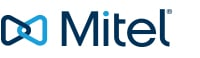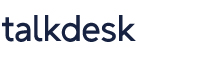### The 5 Must-Haves for CX Management in 2020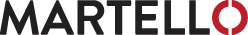### Unleash the Power of 5G and Enhance Your SD-WAN Experience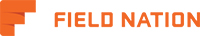### Setting Hourly Rates for Field Service Technicians### The Future of Healthcare### Trisys Delivers on Call Recording## LATEST VIDEOS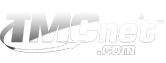### Technology Marketing Corporation

2 Trap Falls Road Suite 106, Shelton, CT 06484 USA
Ph: +1-203-852-6800, 800-243-6002